2019-10-05 22:13:42 lai1170137052 阅读数 148
• ###### 温度传感器DS18B20-第2季第1部分

本课程是《朱有鹏老师单片机完全学习系列课程》第2季第1个课程，主要讲解单片机系统中常用的温度传感器DS18B20。本课程的目标是让大家进一步掌握时序的分析和编程实现，学会移植和调试DS18B20的程序，能够读取温度。

2182 人正在学习 去看看 朱有鹏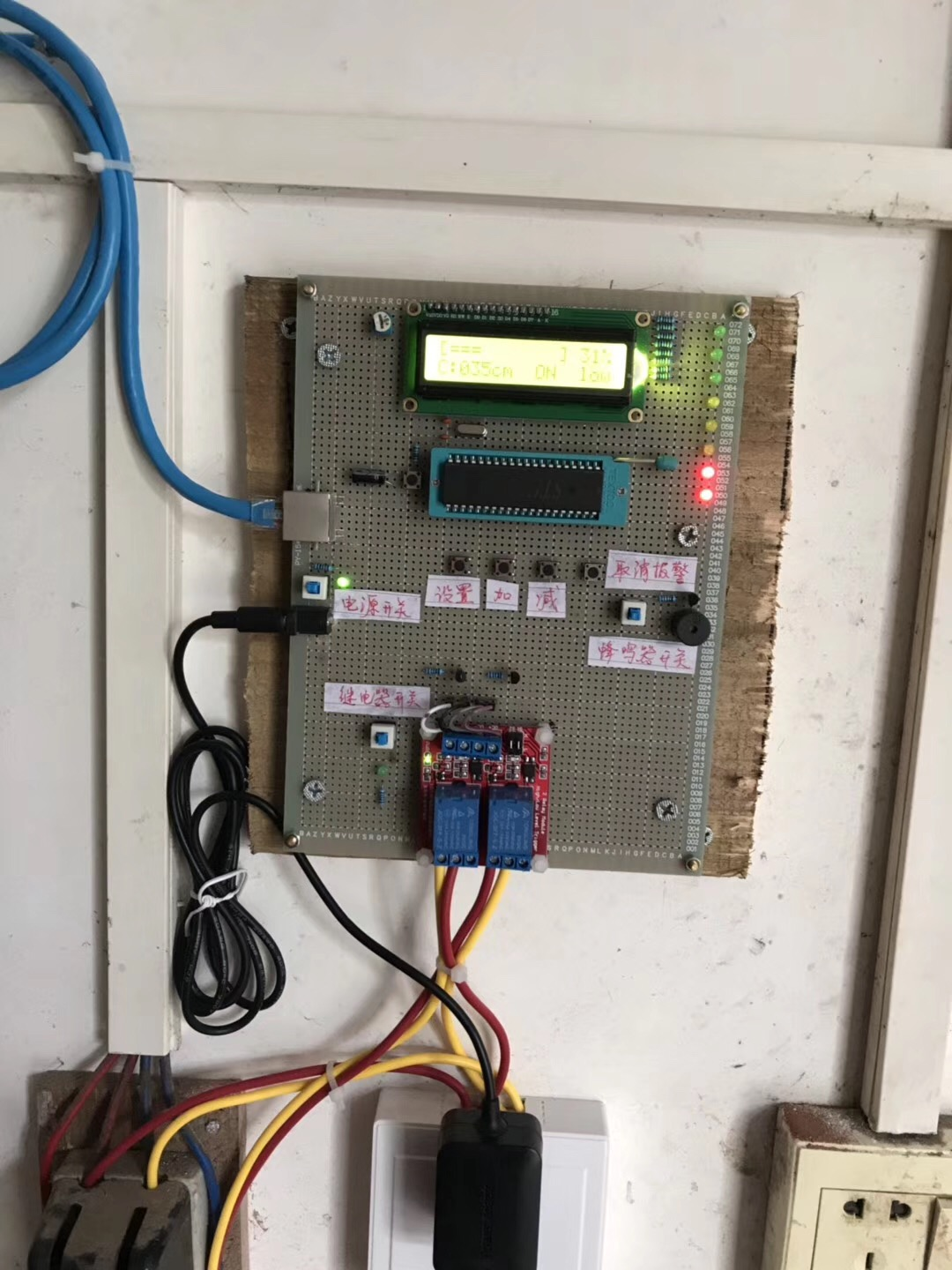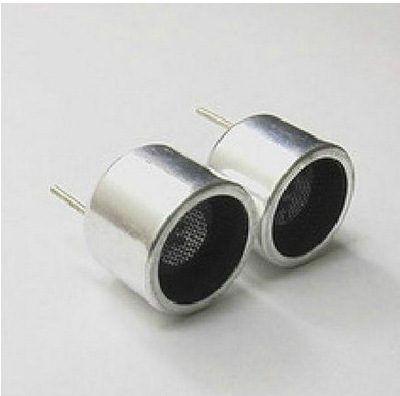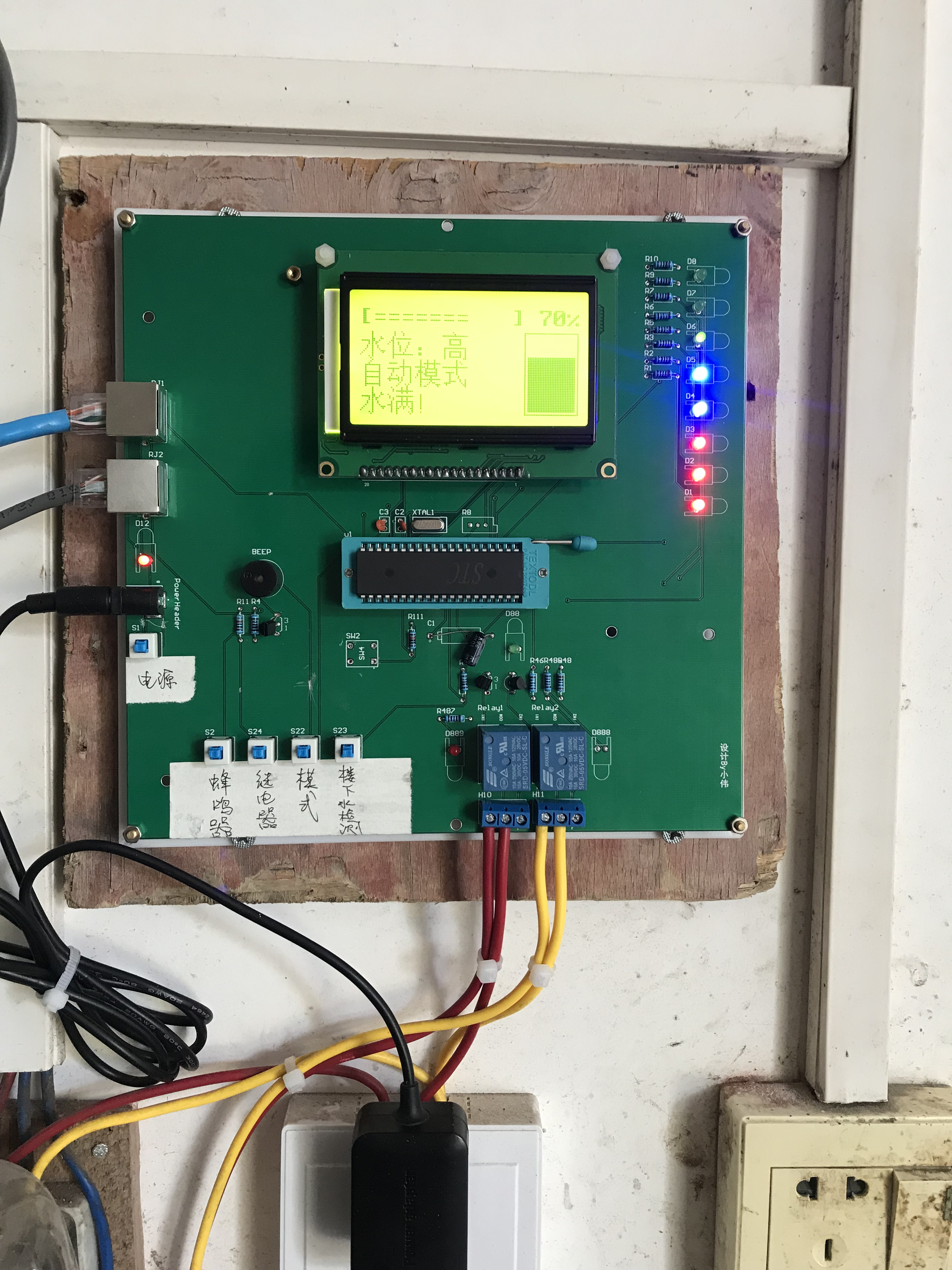``````#include <reg52.h>
#include <string.h>
#include <delay.h>
#include <lcd12864.h>
#include <relay.h>
#include <buzzer.h>
#include <led.h>
#include <sensor.h>

unsigned int percent = 0;// 当前水位与最高水位的百分比
unsigned int buzzer_count = 0;// 用于抽水时蜂鸣器叫计数
unsigned int buzzer_warning_count = 0;// 用于报警蜂鸣器叫计数
unsigned int buzzer_high_warning_max_count = 0;// 用于记录超高报警提示次数
unsigned int buzzer_high_warning_count = 0;// 用于水位超高时报警蜂鸣器叫计数
unsigned int buzzer_low_warning_count = 0;// 用于水位超低时报警蜂鸣器叫计数
unsigned int auto_high = 90;// 设定自动模式最高水位百分比
unsigned int auto_low = 50;// 设定自动模式最低水位百分比
unsigned int manual_high = 90;// 设定手动模式最高水位百分比
unsigned int manual_low = 10;// 设定手动模式最低水位百分比
unsigned int low_count = 0;// 自动模式下，进入低于最低水位次数，防止液面抖动
unsigned int high_count = 0;// 自动模式下，进入低于最高水位次数，防止液面抖动

// 初始化
void init_sensor()
{
P2 = 0x00;// p2口初始化为低电平
check = 1;// 是否检测楼下水池水位开关，高电平不检查
has_water = 0;// 楼下是否有水标志
mode = 1;// 是否是自动抽水模式，0-是；1-否，默认不开启
}

// 计算当前水位的百分比
void calculate_percent()
{
unsigned char current = P2;
unsigned int delayms = 500;// 延时检测时间，11.0592M晶振下
is_warning = 0;// 初始化报警状态
warning_type = 0;// 初始化报警类型

// 计算百分比
if(s7 == 0) {
delaynms(delayms);// 延时检测，防止液面抖动
if(s7 == 0) {// 如果真的等于0
percent = 0;
}
}
if(s7 == 1) {
delaynms(delayms);// 延时检测，防止液面抖动
if(s7 == 1) {
percent = 10;
}
}
if(s6 == 1) {
delaynms(delayms);// 延时检测，防止液面抖动
if(s6 == 1) {
percent = 20;
}
}
if(s5 == 1) {
delaynms(delayms);// 延时检测，防止液面抖动
if(s5 == 1) {
percent = 30;
}
}
if(s4 == 1) {
delaynms(delayms);// 延时检测，防止液面抖动
if(s4 == 1) {
percent = 50;
}
}
if(s3 == 1) {
delaynms(delayms);// 延时检测，防止液面抖动
if(s3 == 1) {
percent = 70;
}
}
if(s2 == 1) {
delaynms(delayms);// 延时检测，防止液面抖动
if(s2 == 1) {
percent = 90;
}
}
if(s1 == 1) {
delaynms(delayms);// 延时检测，防止液面抖动
if(s1 == 1) {
percent = 100;
}
}

// 计算百分比
//		if(current == 0x80) {
//			percent = 100;
//		} else if(current == 0xc0) {
//			percent = 90;
//		}  else if(current == 0xe0) {
//			percent = 70;
//		} else if(current == 0xf0) {
//			percent = 50;
//		} else if(current == 0xf8) {
//			percent = 30;
//		} else if(current == 0xfc) {
//			percent = 20;
//		} else if(current == 0xfe) {
//			percent = 10;
//		} else if(current == 0xff) {
//			percent = 0;
//		} else {
//			// 探测器异常
//			percent = 0;
//			is_warning = 1;
//			warning_type = 1;
//			if(buzzer_warning_count++ >= 20)// 1s * 20 约20s
//			{
//				buzzer_warning_count = 0;
//				buzzer_warning_tips();// 蜂鸣器报警叫5声，led闪烁
//			}
//			relay_stop();// 如果在抽水过程中出异常，关闭继电器
//		}

// 如果没有报警且开启了自动抽水模式
if(mode == 0) {
if(is_warning == 0) {
if(percent <= auto_low) {// 如果低于设定的最低水位
if(low_count++ >= 10) {// 如果进来10次，说明水位是真的到了最低水位，防止液面抖动触发继电器情况

// 是否需要检测楼下水位，为了没有安装楼下水位探测器也能正常使用的情况
if(check == 0) {// 需要
if(has_water == 1) {// 有水
relay_start();// 开启继电器
// low_count = 0;
} else {
is_warning = 1;
warning_type = 2;// 开启液晶显示缺水错误提示
relay_stop();// 如果在抽水过程中没有水了，关闭继电器

//											if(buzzer_warning_count++ >= 20)// 1s * 20 约20s
//											{
//												buzzer_warning_count = 0;
//												buzzer_warning_tips();// 蜂鸣器报警叫5声，led闪烁
//											}
}
} else {//不需要检测楼下水位
relay_start();// 开启继电器
}
}
} else {
low_count = 0;
}
if(percent >= auto_high) {
if(high_count++ >= 3) {// 如果进来3次，说明水位是真的到了最低水位，防止液面抖动触发继电器情况
relay_stop();// 关闭继电器
high_count = 0;
}
} else {
high_count = 0;
}

// 如果继电器已开启
if(relay_open_flag == 1 && is_warning == 0) {
if(buzzer_count++ >= 5)// 每1s进来一次，5s
{
buzzer_count = 0;
buzzer_nms(300);// 蜂鸣器叫300ms左右，提示正在抽水
}
relay_led = 0;// 继电器抽水提示led灯闪烁
delaynms(100);
relay_led = 1;
}
}
} else {// 手动模式下
relay_stop();// 如果突然变为手动模式，若开启继电器，关闭继电器

if(percent <= manual_low) {// 如果低于设定的最低水位，提示抽水
if(buzzer_low_warning_count++ >= 5*60)// 每1s进来一次，5*60s=5min
{
buzzer_low_warning_count = 0;
buzzer_warning_tips();// 蜂鸣器报警叫5声，led闪烁
}
}
if(percent >= manual_high) {// 如果低于设定的最高水位，提示关水
if(buzzer_high_warning_count++ >= 5)// 每1s进来一次，5s
{
buzzer_high_warning_count = 0;
if(buzzer_high_warning_max_count++ < 5) {// 如果超过提醒次数，则不再提醒
buzzer_warning_tips();// 蜂鸣器报警叫5声，led闪烁
}
if(buzzer_high_warning_max_count > 10) {// 防止数据溢出
buzzer_high_warning_max_count = 10;
}
}
} else {
buzzer_high_warning_max_count = 0;// 恢复报警次数
}
}
}

// 根据探测到的距离，计算水位，返回是否超出范围：1-超出；0-没有超出
void calculate_water_level()
{
calculate_percent();// 计算水位百分比
lcd_show_percent();// 液晶显示水位
led_show_percent();// led显示水位
}

``````

2020-01-04 22:05:57 weixin_45380951 阅读数 12
• ###### 温度传感器DS18B20-第2季第1部分

本课程是《朱有鹏老师单片机完全学习系列课程》第2季第1个课程，主要讲解单片机系统中常用的温度传感器DS18B20。本课程的目标是让大家进一步掌握时序的分析和编程实现，学会移植和调试DS18B20的程序，能够读取温度。

2182 人正在学习 去看看 朱有鹏

### Water Sensor水位传感器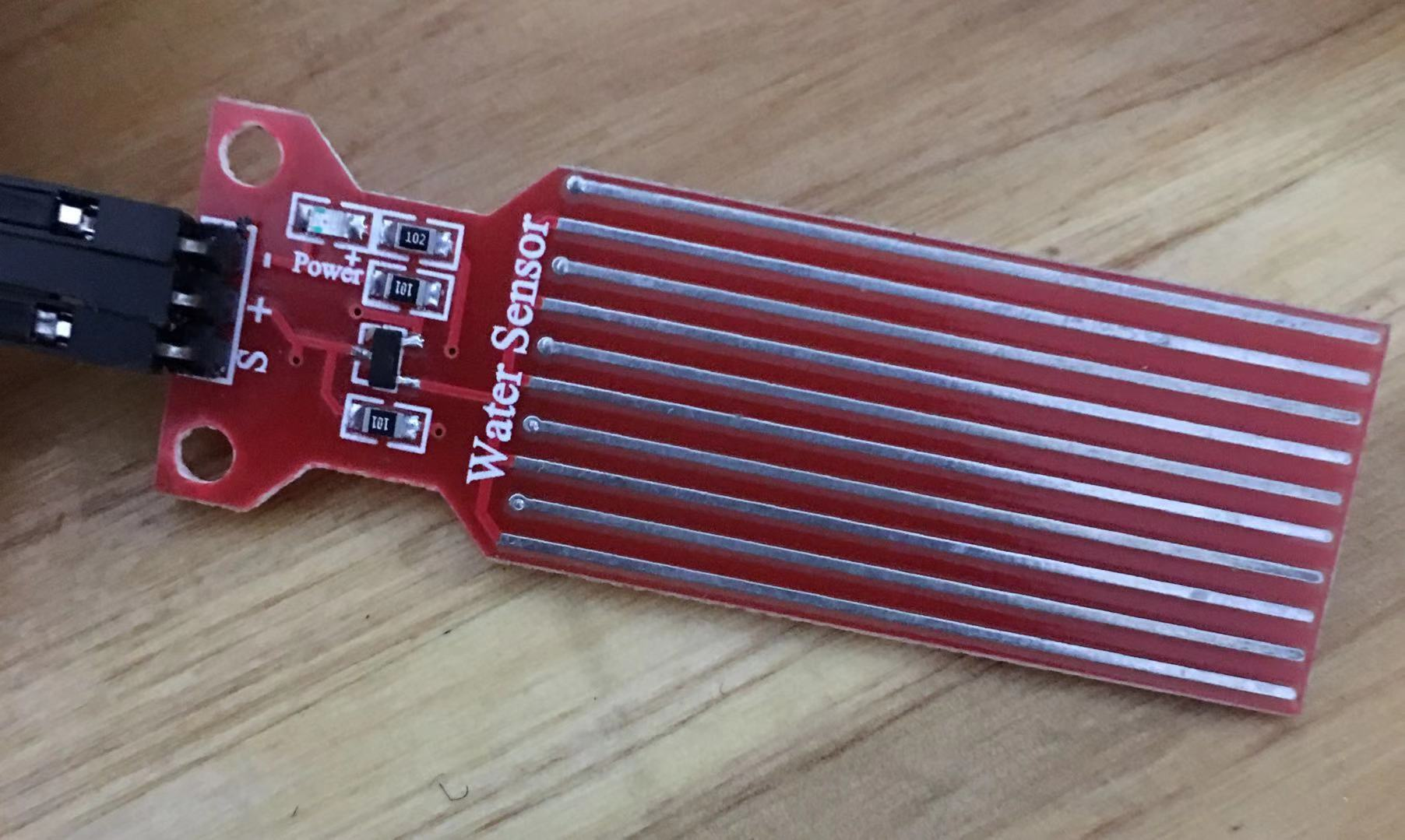#### 特点

1、水量到模拟量的转换
2、可塑性强，本传感器输出为基础模拟值
3、低功耗，灵敏度高
4、可直接与魏处理器或其他逻辑电路相连接，适合各种开发板和控制器（如：Arduino控制器、STC单片机、AVR单片机等）

#### 规格参数

2019-10-11 17:26:55 qq_42377696 阅读数 118
• ###### 温度传感器DS18B20-第2季第1部分

本课程是《朱有鹏老师单片机完全学习系列课程》第2季第1个课程，主要讲解单片机系统中常用的温度传感器DS18B20。本课程的目标是让大家进一步掌握时序的分析和编程实现，学会移植和调试DS18B20的程序，能够读取温度。

2182 人正在学习 去看看 朱有鹏

``````#include <reg51.h>
unsigned char code table[]={0xde,0x91,0xcd,0xd5,0x93,0x2b,0x5f,0xd4,0xdf,0xd1};
sbit sw6=P1^5;//水位6
sbit sw5=P1^4;//水位5
sbit sw4=P1^3;//水位4
sbit sw3=P1^2;//水位3
sbit sw2=P1^1;//水位2
sbit sw1=P1^0;//水位1
sbit beep=P1^6;//蜂鸣器
sbit dianji=P1^7;//电机
sbit LED=P2^6;//电机指示灯
sbit red=P2^3;//red
sbit green=P2^4;//green
sbit yellow=P2^5;//yellow

void delay02s(void)
{
unsigned char i,j,k;
for(i=100;i>0;i--)
for(j=100;j>0;j--)
for(k=248;k>0;k--);
}
main()
{
P0=0;
while(1)
{
if(sw6==0&&sw5==1&&sw4==1&&sw3==1&&sw2==1&&sw1==1)//水位6
{
dianji=0;
LED=0;red=0;green=0;yellow=0;
beep=0;
P0=table;
delay02s();
}

if(sw6==1&&sw5==0&&sw4==1&&sw3==1&&sw2==1&&sw1==1)//水位5
{
dianji=0;
LED=0;red=0;green=0;yellow=0;
beep=0;
P0=table;
}

if(sw6==1&&sw5==1&&sw4==0&&sw3==1&&sw2==1&&sw1==1)//水位4
{
dianji=0;
LED=0;red=0;green=0;yellow=0;
beep=0;
P0=table;
}

if(sw6==1&&sw5==1&&sw4==1&&sw3==0&&sw2==1&&sw1==1)//水位3
{
dianji=0;
LED=0;red=0;green=1;yellow=1;
beep=0;
P0=table;
}

if(sw6==1&&sw5==1&&sw4==1&&sw3==1&&sw2==0&&sw1==1)//水位2
{
dianji=1;
LED=1;red=1;green=1;yellow=0;
beep=1;
P0=table;
}

if(sw6==1&&sw5==1&&sw4==1&&sw3==1&&sw2==1&&sw1==0)//水位1
{
dianji=1;
LED=1;red=1;green=0;yellow=1;
beep=1;
P0=table;
}
}

}
``````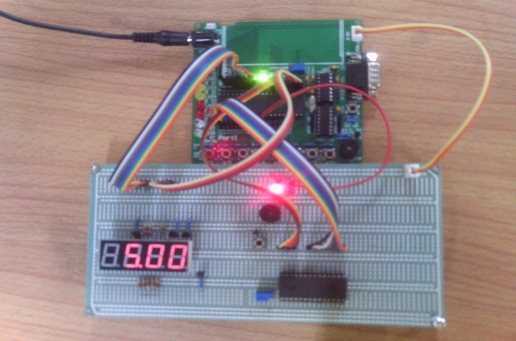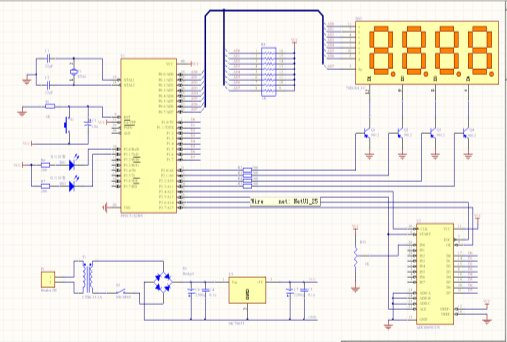2019-04-07 01:40:41 huazhen1234 阅读数 1704
• ###### 温度传感器DS18B20-第2季第1部分

本课程是《朱有鹏老师单片机完全学习系列课程》第2季第1个课程，主要讲解单片机系统中常用的温度传感器DS18B20。本课程的目标是让大家进一步掌握时序的分析和编程实现，学会移植和调试DS18B20的程序，能够读取温度。

2182 人正在学习 去看看 朱有鹏

1）检测鱼塘水位；水位过高闸门开启放水，水位过低关闭闸门。
2）检测是否有人靠近鱼塘，如果有人靠近，蜂鸣报警+短信报警，要区分路过和真正的靠近。

1）单片机最小系统板：STM32F103C8T6
2）开发环境：MDK5.14
3）库函数：标准库V3.5
4）热释电红外传感器：HC-SR501
5）电机：5V电机
6）短信模块：SIM800C
7）水位传感器：光电式水位传感器
8）蜂鸣器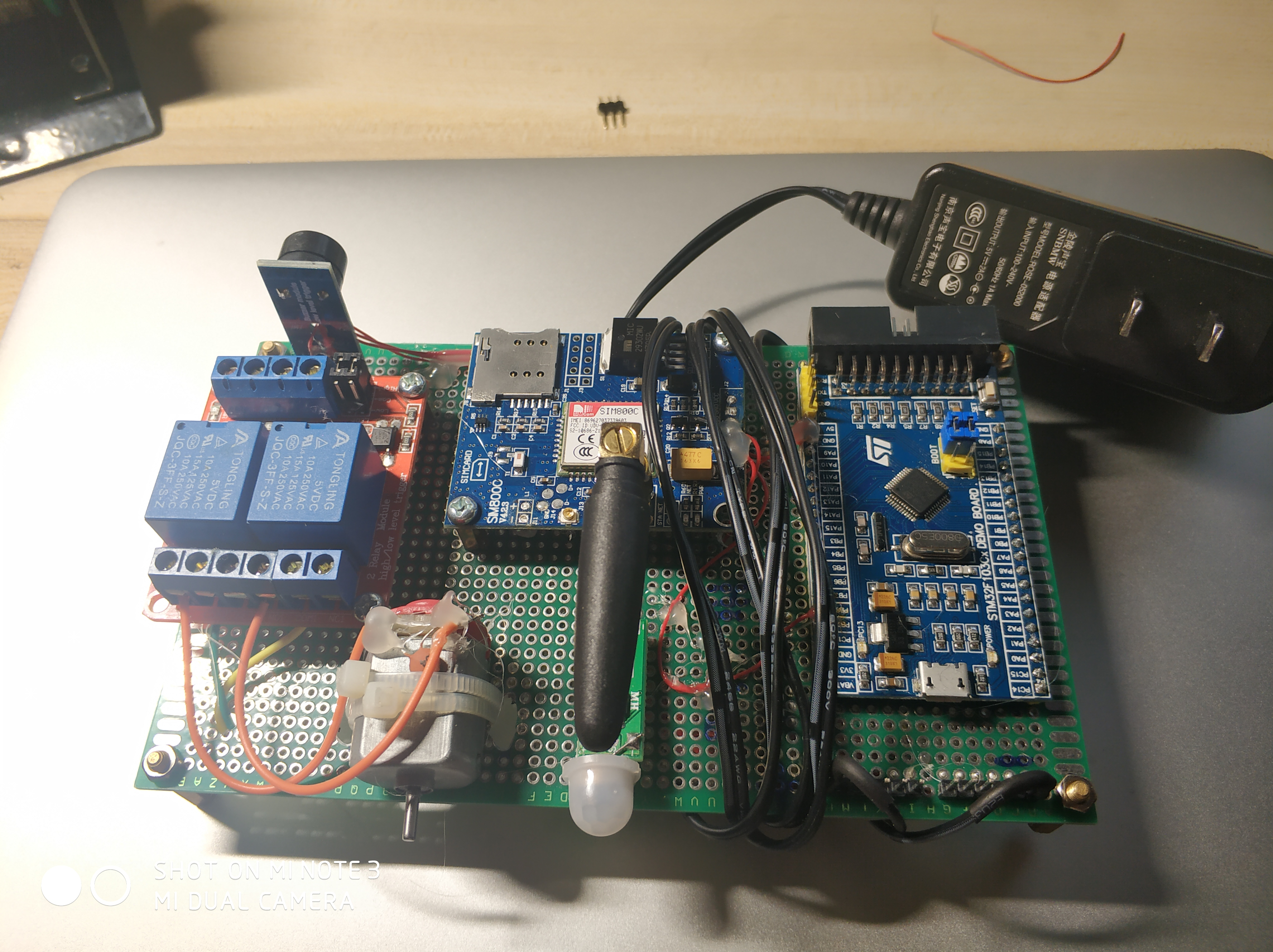1）电机驱动：驱动继电器控制电机转动；
2）蜂鸣器驱动：PWM
3）SIM800C驱动：USART
4）液位传感器驱动：IO
5）热释电红外传感器驱动：IO，TIM

2017-04-21 15:16:09 zhang_zhonghai 阅读数 223
• ###### 温度传感器DS18B20-第2季第1部分

本课程是《朱有鹏老师单片机完全学习系列课程》第2季第1个课程，主要讲解单片机系统中常用的温度传感器DS18B20。本课程的目标是让大家进一步掌握时序的分析和编程实现，学会移植和调试DS18B20的程序，能够读取温度。

2182 人正在学习 去看看 朱有鹏

②控制加热时间，加热温度，水位（按键控制）。【范围0-99】

③显示水位，水温，加热时间，设定水位，水温（LCD1602显示）。

④报警系统，水温到达预定值报警。

⑤水位低于最小值，自动加水。

⑥通过电磁继电器控制加热模块和加水模块（加热加水模块，电压220V）。